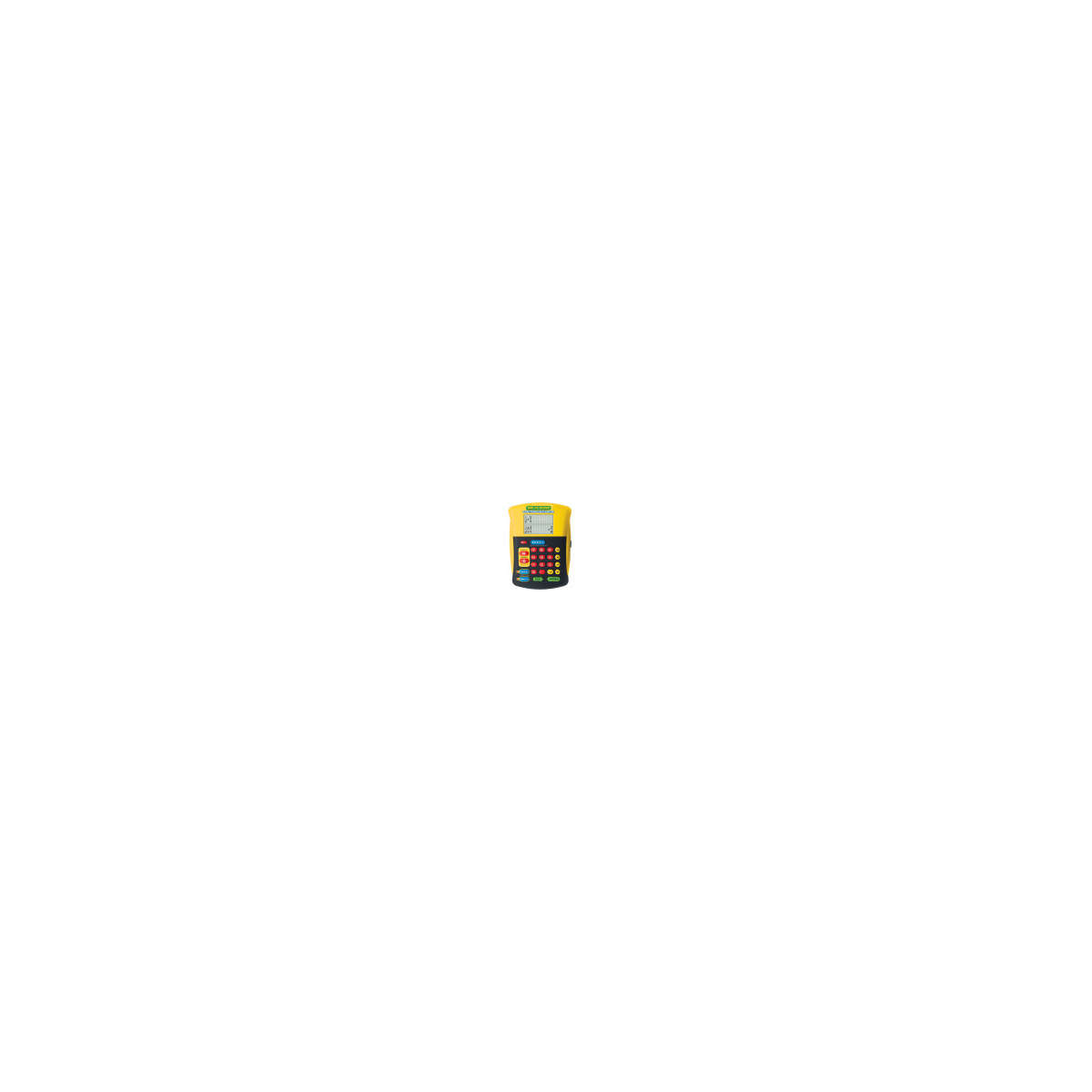•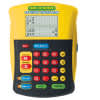•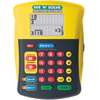•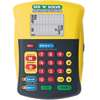•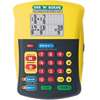•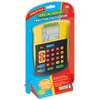# See 'n' Solve Fraction Calculator

Product Number: EI-8479
Let this unique calculator model solving fraction problems step-by-step. Oversize LCD screen displays each step, shows amount...
Quantity

Let this unique calculator model solving fraction problems step-by-step. Oversize LCD screen displays each step, shows amounts in mixed number and improper fraction form, and answers in reduced form. Easily input addition, subtraction, multiplication and division equations or convert between fractions, decimals and percents. Also features normal calculator mode. Includes 12-page Activity Guide and quick reference stickers. Measures approximately 4 1/2"L x 6 1/4"H. Requires 2 AA batteries, not included. Grades 3+
EI-8479